Back to Pumps

The Condensation Effect on Liquid Ring Vacuum Pumps

August 22nd, 2015 Posted by Dane CME

Under certain conditions the liquid ring vacuum pump capacity can be increased substantially for a given speed and power consumption.  This article is written as a technical overview of the thermodynamics behind this windfall in power savings.

The liquid ring vacuum pump is a constant volume device, which means that at a given speed the volume of gas taken in will be a constant. If the gas steam coming to the vacuum pump contains a condensable vapor, and the vapor is condensed before it is drawn into the bucket of the liquid ring pump, the gas will occupy less space than it had before the gas was condensed. Since the pump draws in a constant volume, a measurement of the flow before condensation (this is what you care about, the flow at your filter or couch roll or uhle box, for instance) will be higher than the capacity of the vacuum pump as stated in the pump curves.

Before we get into the explanation of how this happens, let’s look at an example. A saturated mixture (100% humidity) of water vapor and air at 100°F and 20”Hg vacuum will be 19.47% water vapor by volume; the rest is air. A saturated mixture (100% humidity) of water vapor and air at 80°F will be 10.40% water vapor by volume; the rest is air. So if we have one cubic foot of air at 100°F it will be .8053 cubic feet of air and .1947 cubic feet of water vapor. If we cool this mixture to 80°F without changing the vacuum level we will have .7765 cubic feet of air (the volume decreases because the temperature decreases) but only .0901 cubic feet of water vapor (the volume decreases with the temperature decrease but also because the water vapor loses 47% to condensation) for a total of .8666 cubic feet. To get back to the one cubic foot, we have to add .1344 cubic feet so the capacity percentage increase is 15.5%.  Since you are paying for CFM per horsepower, this is a bonus; and almost for free.

So let’s look at the science. If you put a liquid in an evacuated closed chamber some of the liquid will evaporate until equilibrium is reached between the gaseous phase (vapor) and the liquid phase. Since the chamber is closed the higher amount of gas will be evidenced by a higher pressure. This pressure is called the saturated vapor pressure. The saturated vapor pressure increases as the temperature rises. The attached graph shows the saturated vapor pressure of water versus temperature.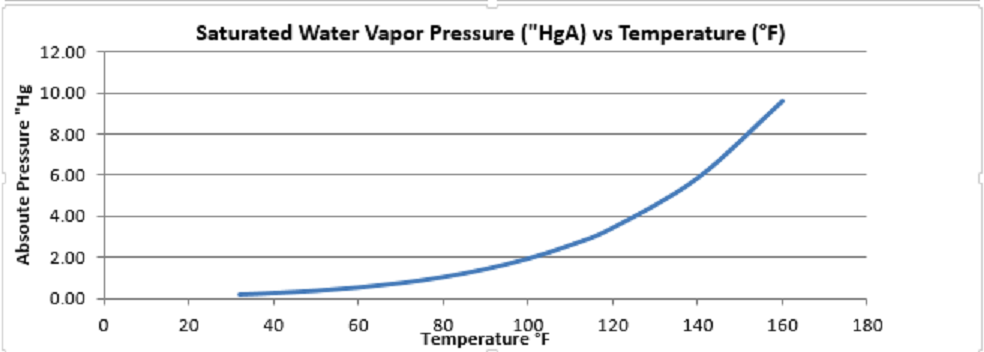For a mixture of gases each gas will occupy the same volume and the pressure of the mixture will be the sum of the pressures that each gas would be at if it alone occupied that volume. This individual pressure is called the partial pressure of the individual gas. This is Dalton’s Law. So if you have a mixture of air and water vapor, the water vapor can be at any pressure up to the saturation pressure at the temperature of the mixture. Above that pressure the water vapor will turn to water and the vapor pressure will remain constant. Also, if liquid water is present, the minimum pressure of the mix can be no lower than the vapor pressure of the water vapor, because at this point the gas is all water vapor, there is no air.

In our example above the incoming gas mixture was at 100°F, so the partial pressure of the water vapor from the graph will be 1.93”Hg. The vacuum level is 20 “Hg, so the absolute pressure is 29.92 – 20 =9.92 “Hg. The pressure of the air is thus  9.92 – 1.93 = 7.99 “Hg. Since this is a gas at low pressure, it will follow ideal gas relationships which means the volume % of the individual gases will be the same as the pressure %. So for our mix of saturated water vapor and air we have 1.93/9.92=19.47% water vapor and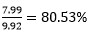air. If we have 1 cubic foot of mixture, we will have .8053 cubic feet of air and .1947 cubic feet of water vapor.

If we now cool the mixture to 80°F, the air will decrease in volume by the ratio of absolute temperature, orcubic feet. The water at 80°F will have a saturated vapor pressure of 1.03”Hg or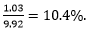Applying this percentage to the .7765 cubic feet we find the water vapor is .0901 cubic feet. The total volume of the mixture is thus  .7765 + .0901 =.8666. Since we want one cubic foot, we need to add .1334 cubic feet or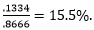This is the volume increase because of temperature drop and condensation. So you can run the vacuum pump 15.5% slower and save 21.7% on horsepower than you would if you did not have the condensation effect.The chart below shows the percentage gains at some other temperatures.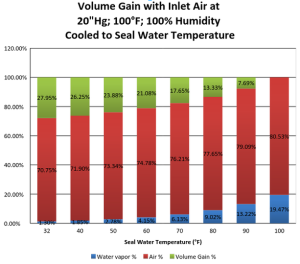This gain in capacity and decrease in power is almost free. The cost is an increase in the heat produced by the vacuum pump. The heat increase is equal to the energy released when the water condenses. The energy released heats the sealing liquid. This can be an issue depending upon the cost of the sealing fluid and its treatment if it is recirculated.  In our pump tests in the Pacific Northwest where the humidity is usually around 50% and there is no condensing effect, the seal water temperature rise across the pump is usually about 7°F. For our customers running paper machines with air at 100°F and 100% humidity, the temperature rise can be as much as 30°F.

The above example is clean and theoretical; in actual practice other factors enter the equation. Be aware that some manufacturers have published curves that include the condensation effect, therefore increasing the stated capacity by about 10%. We do not do this as we calculate each case individually and are transparent with the customer in the pump size we recommend for their application and explain all of the assumptions that we make. Call us with your questions, we like to solve your problems.